•5星
135.91MB qq_34351067 2021-04-16 14:11:28
•5星
880KB weixin_42696271 2021-09-11 13:05:15
•5星
326KB weixin_42120750 2021-04-26 16:06:08
•5星
353KB qq_41934573 2021-06-24 22:06:26
•4星
1.54MB qq_41934573 2021-05-19 13:39:28
•5星
9KB weixin_42696271 2021-09-11 14:50:31
•5星
1KB weixin_47505475 2021-04-11 17:43:30
•5星
9KB weixin_42696271 2021-09-11 02:49:23
•5星
2KB weixin_42676876 2021-10-01 11:05:21
•5星
15.41MB weixin_42696333 2021-09-10 20:51:04
• Matlab建立FP-Tree Matlab树结构 Matlab FPTree 树结构

安利一下Matlab用于查询函数用法的官网https://ww2.mathworks.cn/help/matlab/ 一、要求 给定2013,2014,2015,2016,2017的福彩双色球数据，共767期数据 (1) 使用一种编程语言实现FP-tree的建树算法，并...

目录

一、要求

二、数据格式

三、数据结构

四、Matlab代码

main.m 主函数

insertChild.m 【递归】插入子树

isChild.m 判断该节点是否是子树，并返回位置

nodenumber.m文件 【递归】寻找当前树的叶子节点个数

nodemax.m函数  【递归】寻找节点数最多的路径

supportmax.m函数 【递归】寻找支持度最大的路径

demo 简短的方便查看结果的样例代码

五、数据和代码获取

安利一下Matlab用于查询函数用法的官网MATLABDocumentation- MathWorks 中国

一、要求

给定2013,2014,2015,2016,2017的福彩双色球数据，共767期数据
(1) 使用一种编程语言实现FP-tree的建树算法，并应用于此数据构建FP-tree；
(2) 统计所建FP-tree中的节点数目，包括根节点；
(3) 通过使用一个算法给出从根到叶子的节点数最多一条路径，并显示其中除根以外每个节点上的支持度计数;
(4) 给出关于根到叶子的节点一条路径，且该路径上所有节点的支持度计数之和最大，显示每个节点上的支持度计数及总和。

二、数据格式

示例：

期号       红球1    红球2    红球3    红球4    红球5    红球6

2013001    6          8           14         15          24         25
2013002    1         16          18         22          28         30
2013003    22       23          26         27          28         33
2013004    6         10          16         20          27         32

三、数据结构

用一个1*n的cell来存放一个节点的信息

cell{1,1}储存当前节点所对应的球编号，cell{1,2}储存当前节点的支持度计数，cell{1,m}(2<m<=n)指向该节点下的子节点

如图所示为根节点的节点信息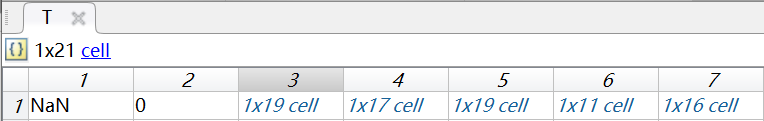四、Matlab代码

main.m 主函数

clear
%% 主程序
%% 读取数据
ball=importdata('作业01-双色球数据ssq2013_2017.txt');
ball=ball.data(:,2:end);%提取有效数据 得到767*6的矩阵
%%
f=tabulate(ball(:));
f=sortrows(f,-2);%按支持度降序排列
%项头表，第一列是球编号，第二列是支持度，第三列是占总体的比例
%% 设置支持度阈值
max=f(1,2);%支持度最大值
threshold=max*0.8;%支持度阈值
d=f(f(:,2)<=threshold,1);%提取出支持度低于阈值的球编号至d
f(f(:,2)<=threshold,:)=[];%删去支持度低于阈值的球编号
order=f(:,1);%排列顺序
for i=1:length(ball)
[~,index]=ismember(ball(i,:),order);
temp=[ball(i,:);index];
temp(:,temp(2,:)==0)=NaN;
temp=sortrows(temp',2)';
ball(i,:)=temp(1,:);
end
%% 初始化根节点
T{1,1}=NaN;
T{1,2}=0;
%% 建立FP树
for i=1:length(ball)
temp=ball(i,:);%temp为当第i行数据
temp=temp(~isnan(temp));%删去NaN
if ~isempty(temp)%temp不空，向T中插入temp
T=insertChild(T,temp,1);
end
end
%% 统计节点数目，包括根节点
Node_number=nodenumber(T);

%% 统计节点最多的路径，并记录其每个节点的支持度
% 第一行为球的编号 第二行为编号对应的支持度
% 编号NaN表示根节点
Node_max=nodemax(T);
%% 节点支持度之和最大的路径
Node_support=supportmax(T);

insertChild.m 【递归】插入子树

function cell=insertChild(cell,num,k)
loc=isChild(cell,num(k));
if loc~=0%该元素是子树
cell{1,loc}{1,2}=cell{1,loc}{1,2}+1;
if k~=length(num)
cell{1,loc}=insertChild(cell{1,loc},num,k+1);
end
else %该元素非子树
if k==length(num)
child{1,1}=num(k);
child{1,2}=1;
cell{1,length(cell)+1}=child;
else
len=length(cell);
cell{1,len+1}{1,1}=num(k);
cell{1,len+1}{1,2}=1;
cell{1,len+1}=insertChild(cell{1,len+1},num,k+1);
end
end
end

isChild.m 判断该节点是否是子树，并返回位置

function loc=isChild(cell,num)
%loc表示该元素在cell中的位置
loc=0;
if length(cell)>2
for i=3:length(cell)
if isempty(cell{1,i})
break
elseif cell{1,i}{1,1}==num
loc=i;
break;
end
end
end
end

nodenumber.m文件 【递归】寻找当前树的叶子节点个数

function y=nodenumber(T)
len=length(T);
if len==2%当前节点为叶子节点
y=1;
else
y=1;
for i=3:len
y=y+nodenumber(T{1,i});
end
end
end

nodemax.m函数  【递归】寻找节点数最多的路径

function y=nodemax(T)
len=length(T);
if len==2%当前节点为叶子节点
y=[T{1,1};T{1,2}];
else
k=[T{1,1};T{1,2}];
max=1;
for i=3:len
temp=[k nodemax(T{1,i})];
if length(temp)>max
max=length(temp);
y=temp;
end
end
end
end

supportmax.m函数 【递归】寻找支持度最大的路径

function y=supportmax(T)
len=length(T);
if len==2%当前节点为叶子节点
y=[T{1,1};T{1,2}];
else
k=[T{1,1};T{1,2}];
max=0;
for i=3:len
temp=[k supportmax(T{1,i})];
if sum(temp(2,:))>max
max=sum(temp(2,:));
y=temp;
end
end
end
end

demo 简短的方便查看结果的样例代码

ball=[2 7 5;
3 6 1;
7 5 3;
6 2 3;
7 4 5];
threshold=2;%支持度阈值
f=tabulate(ball(:));        %编号  支持度
f=sortrows(f,-2);           %  3      3
d=f(f(:,2)<threshold,1);    %  5      3
f(f(:,2)<threshold,:)=[];   %  7      3
order=f(:,1);               %  2      2
%  6      2
%  1      1    （低于阈值）
%  4      1    （低于阈值）
%%
for i=1:length(ball)                    % 去除支持度低于2的元素后，按支持度对每行ball重排
[~,index]=ismember(ball(i,:),order);% [5,7,2;
temp=[ball(i,:);index];             %  3,6,NaN;
temp(:,temp(2,:)==0)=NaN;           %  3,5,7;
temp=sortrows(temp',2)';            %  3,2,6;
ball(i,:)=temp(1,:);                %  5,7,NaN]
end
%% 初始化根节点
T{1,1}=NaN;
T{1,2}=0;
%% 建立FP树                                   %%      所得树
for i=1:length(ball)                              %         NaN
temp=ball(i,:);%temp为当第i行数据              %        /  \
temp=temp(~isnan(temp));%删去NaN              %       5    3
if ~isempty(temp)%temp不空，向T中插入temp      %       |  / | \
T=insertChild(T,temp,1);                 %       7 6  5  2
end                                          %       |    |  |
end                                              %       2    7  6
%% 结果输出
%统计节点数目，包括根节点
Node_number=nodenumber(T);
% 统计节点最多的路径，并记录其每个节点的支持度
% 第一行为球的编号 第二行为编号对应的支持度
% 编号NaN表示根节点
Node_max=nodemax(T);
% 节点支持度之和最大的路径
Node_support=supportmax(T);

五、数据和代码获取

关注公众号，回复“FP树”即可获取数据和全部代码。展开全文qq_42276781 2020-01-13 22:32:29
• 792KB weixin_38679449 2021-05-27 10:35:20
• 769KB weixin_38692928 2021-06-01 17:22:44
• 很久不写博客了，感觉很长一段时间只是一味的看书，疏不知一味地看书、写代码会导致自己的思考以及总结能力变得衰弱。...决策是一种对实例分类的结构中包含叶子节点与内部节点。内部节点主要是数据中的某一特

很久不写博客了，感觉很长一段时间只是一味的看书，疏不知一味地看书、写代码会导致自己的思考以及总结能力变得衰弱。所以，我决定还是继续写博客。废话不多说了，今天想主要记录数据挖掘中的决策树。希望能够将自己的理解写得通俗易懂。

决策树是一种对实例分类的树形结构，树中包含叶子节点与内部节点。内部节点主要是数据中的某一特性，叶子节点是根据数据分析后的最后结果。

先看一组数据：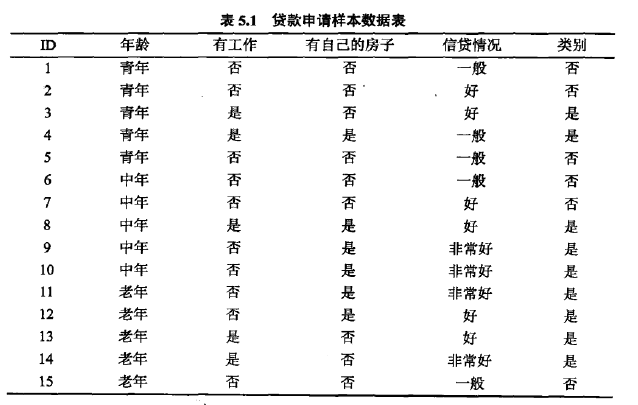这组数据的特性包含年龄、工作与否、是否有房、信贷情况以及最终分类结果贷款是否成功，共包含15组样例。

构建数的形状可以有多种，如下：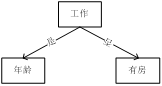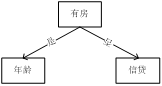如果随意构建树，那将会导致有的构建树比较庞大，分类时代价比较大，有的构建树比较小，分类代价小。

比如针对是否有房这一列，发现如果样本有房这一列为是，最终分类结果便是可以贷款，而不需要判断其他的特性，便可以获得最终部分分类结果。

因此，构建树需要以最小的代价实现最快的分类。根据何种标准进行判别呢？

在信息论与概率统计中，熵是表示随机变量不确定的量度，设x是一个取有限个值的离散随机变量，其概率分布为：则随机变量x的熵定义为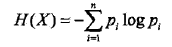熵越大，其不确定性越大。

随机变量x在给定条件y下的条件熵为H(y|x);

信息增益表示得知特征x的信息而使得y类信息的不确定减少的程度。

因此，特征A对训练集D的信息增益g(D，A)，定义为集合D的熵H(D)与特征A给定条件下D的条件熵H(D|A）之差，即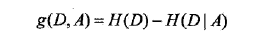对表5.1给定的训练数据集D,计算各特征对其的信息增益，分别以A1,A2,A3,A4表示年龄，有工作，有自己的房子和信贷情况四个特征，则

（1）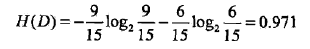（2）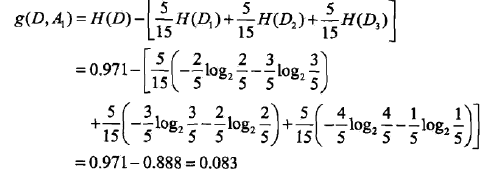这里D1,D2,D3分别是D中A1取为青年、中年、老年的样本子集，同理，求得其他特征的信息增益：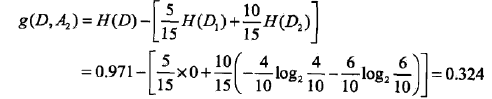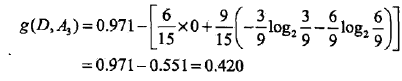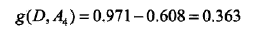接下来根据之前的信息增益，对决策树进行生成，这里主要使用ID3算法，C4.5算法与之类似，只是将信息增益衡量转为信息增益比衡量。

主要方法如下：

从根节点开始，对节点计算所有可能的特征的信息增益，选择信息增益最大的特征作为该节点的特征，由该特征的不同取值建立子节点，再对子节点递归调用以上方法，构建决策树。

那么递归何时停止呢？当训练集中所有实例属于同一类时，或者所有特征都选择完毕时，或者信息增益小于某个阈值时，则停止递归，。

举例来说，根据之前对表5.1的熵的计算，由于A3(是否有自己的房子）信息增益最大，所以以A3为决策树的根节点的特征，它将数据集分为两个子集D1(A3取是）和D2(A3取否），由于D1的分类结果都是可以贷款，所以它成为叶节点，对于D2,则从特征A1,A2,A4这三个特征中重新选择特征，计算各个特征的信息增益：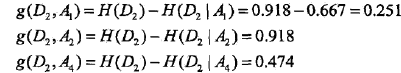因此选择A2作为子树节点，针对A2是否有工作这个特征，根据样本分类结果发现有工作与无工作各自的样本都属于同一类，因此将有工作与无工作作为子树的叶节点。这样便生成如下的决策树：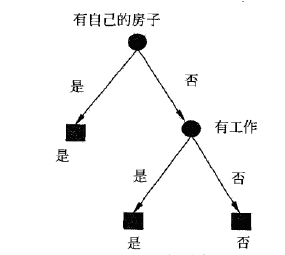决策树生成算法递归的产生决策树，往往对训练数据分类准确，但对未知数据却没那么准确，即会出现过拟合状况。解决这个问题可以通过决策树的剪枝，让决策树简化。本文暂不对决策树的剪枝进行详细描述。

接下来，即对决策树实现的matlab代码：

1、首先，定义决策树的数据结构

tree

{

int pro    //是叶节点（0表示）还是内部节点（1表示）

int value //如果是叶节点,则表示具体的分类结果，如果是内部节点，则表示某个特征

int parentpro //如果该节点有父节点，则该值表示父节点所表示特征的具体属性值

tree  child[]  //表示该节点的子树数组

}

2、根据训练集数据通过递归形成树：

function tree = maketree(featurelabels,trainfeatures,targets,epsino)
tree=struct('pro',0,'value',-1,'child',[],'parentpro',-1);
[n,m] = size(trainfeatures); %where n represent total numbers of features,m represent total numbers of examples
cn = unique(targets);%different classes
l=length(cn);%totoal numbers of classes
if l==1%if only one class,just use the class to be the lable of the tree and return
tree.pro=0;%reprensent leaf
tree.value = cn;
tree.child=[];
return
end
if n==0% if feature number equals 0
H = hist(targets, length(cn)); %histogram of class
[ma, largest] = max(H); %ma is the number of class who has largest number,largest is the posion in cn
tree.pro=0;
tree.value=cn(largest);
tree.child=[];
return
end

pnode = zeros(1,length(cn));
%calculate info gain
for i=1:length(cn)
pnode(i)=length(find(targets==cn(i)))/length(targets);
end
H=-sum(pnode.*log(pnode)/log(2));
maxium=-1;
maxi=-1;
g=zeros(1,n);
for i=1:n
fn=unique(trainfeatures(i,:));%one feature has fn properties
lfn=length(fn);
gf=zeros(1,lfn);
fprintf('feature numbers:%d\n',lfn);
for k=1:lfn
onefeature=find(fn(k)==trainfeatures(i,:));%to each property in feature,,calucute the number of this property
for j=1:length(cn)
oneinonefeature=find(cn(j)==targets(:,onefeature));
ratiofeature=length(oneinonefeature)/length(onefeature);
fprintf('feature %d, property %d, rationfeature:%f\n',i, fn(k),ratiofeature);
if(ratiofeature~=0)
gf(k)=gf(k)+(-ratiofeature*log(ratiofeature)/log(2));
end
end
ratio=length(onefeature)/m;
gf(k)=gf(k)*ratio;
end
g(i)=H-sum(gf);
fprintf('%f\n',g(i));
if g(i)>maxium
maxium=g(i);
maxi=i;
end
end

if maxium<epsino
H = hist(targets, length(cn)); %histogram of class
[ma, largest] = max(H); %ma is the number of class who has largest number,largest is the posion in cn
tree.pro=0;
tree.value=cn(largest);
tree.child=[];
return
end

tree.pro=1;%1 represent it's a inner node,0 represents it's a leaf
tv=featurelabels(maxi);
tree.value=tv;
tree.child=[];
featurelabels(maxi)=[];

%split data according feature
[data,target,splitarr]=splitData(trainfeatures,targets,maxi);
%tree.child=zeros(1,length(data));
%build child tree;
fprintf('split data into %d\n',length(data));
for i=1:length(data)
disp(data(i));
fprintf('\n');
disp(target(i));
fprintf('\n');
end
fprintf('\n');

for i=1:size(data,1)
result = zeros(size(data{i}));
result=data{i};
temptree=maketree(featurelabels,result,target{i},0);
tree.pro=1;%1 represent it's a inner node,0 represents it's a leaf
tree.value=tv;
tree.child(i)=temptree;
tree.child(i).parentpro = splitarr(i);
fprintf('temp tree\n');
disp(tree.child(1));
fprintf('\n');
end
disp(tree);
fprintf("now root tree,tree has %d childs\n",size(tree.child,2));
fprintf('\n');
for i=1:size(data,1)
disp(tree.child(i));
fprintf('\n');
end
fprintf('one iteration ends\n');
end

3、根据某个特征，将数据集分成若干子数据集

function [data,target,splitarr]=splitData(oldData,oldtarget,splitindex)
fn=unique(oldData(splitindex,:));
data=cell(length(fn),1);

target=cell(length(fn),1);
splitarr=zeros(size(fn));
for i=1:length(fn)
fcolumn=find(oldData(splitindex,:)==fn(i));
data(i) =oldData(:,fcolumn);
target(i) = oldtarget(:,fcolumn);
data{i}(splitindex,:)=[];
splitarr(i)=fn(i);
end
end

4、打印决策树

function printTree(tree)
if tree.pro==0
fprintf('(%d)',tree.value);
if tree.parentpro~=-1
fprintf('its parent feature value:%d\n',tree.parentpro);
end
return
end
fprintf('[%d]\n',tree.value);
if tree.parentpro~=-1
fprintf('its parent feature value:%d\n',tree.parentpro);
end
fprintf('its subtree:\n');
childset = tree.child;
for i=1:size(childset,2)
printTree(childset(i));
end
fprintf('\n');
fprintf('its subtree end\n');
end

5、对某个具体的样本进行结果预测

function result=classify(data, tree)
while tree.pro==1
childset=tree.child;
v=tree.value;
for i=1:size(childset,2)
child = childset(i);
if child.parentpro==data(v);
tree=child;
break;
end
end
end
result=tree.value;
end

接下来对数据用代码进行测试

clear all; close all; clc
featurelabels=[1,2,3,4];
trainfeatures=[1,1,1,1,1,2,2,2,2,2,3,3,3,3,3;%each row of trainfeature represent one feature and each column reprensent each examples
0,0,1,1,0,0,0,1,0,0,0,0,1,1,0;
0,0,0,1,0,0,0,1,1,1,1,1,0,0,0;
1,2,2,1,1,1,2,2,3,3,3,2,2,3,1
];
targets=[0,0,1,1,0,0,0,1,1,1,1,1,1,1,0];%represent classification results according to trainfeatures
tree=maketree(featurelabels,trainfeatures,targets,0);
printTree(tree);
data=[2,0,0,1];
result=classify(data,tree);
fprintf('The result is %d\n',result);

关于决策树的原理构建大概就结束了，后期可以继续完成对决策树的剪枝或者将决策树由多叉树转化为二叉树，让决策树更加高效矮小。源码地址：https://github.com/summersunshine1/datamining。

展开全文u013453936 2016-10-23 10:55:09
• Tree data structure as a MATLAB classA tree is a hierarchical data structure where every node has exactly one parent (expect the root) and no or several children.Along with this relational structure, ...

Tree data structure as a MATLAB class

A tree is a hierarchical data structure where every node has exactly one parent (expect the root) and no or several children.

Along with this relational structure, each node can store any kind of data.

This class implements it using plain MATLAB syntax and arrays. Most useful methods are implemented, using overloading of MATLAB functions for tree objects.

For instance you can type:

>> find ( (a.^2 .* b) > (c - 5) & d )

with a, b, c and d being tree objects.

Very handy, trivial to use. A rather long tutorial is included to walk you through trees, and show how to make the best out of them.

展开全文weixin_33946659 2021-04-20 00:50:12
• 46KB weixin_38552536 2021-06-20 07:08:11
• 数据结构：常见数据结构的 MATLAB 实现，包括树、哈希表、堆和列表-matlab开发 matlab

24KB weixin_38645373 2021-06-01 10:45:19
• 28KB weixin_38697171 2021-05-20 13:31:31
• 4.23MB weixin_38677306 2021-06-04 09:09:24
• 981KB weixin_38693311 2021-05-27 10:37:43
• 广度优先遍历树的matlab算法实现 广度优先4星
1KB wyx112 2013-06-11 13:34:07
• 52KB weixin_38660359 2021-06-01 17:22:37
• 在拟线性时间内构建组件树：水平集的连通组件可以组织成树状结构。-matlab开发 matlab

253KB weixin_38588592 2021-05-30 12:59:55
• 故障树的MATLAB程序。 MATLAB

19KB kjx11 2019-04-30 09:22:14
• 127KB weixin_38600432 2021-05-23 13:08:54
• 决策树算法 MATLAB 简单实现 决策树 MATLAB Python

最近在数据挖掘与机器学习的课程上刚刚学到了决策算法，于是，想自己用 MATLAB 简单实现一下。虽然拿其中最简单算法的进行实现，但是，从构思–编写–初步完成，也花费了不少时间，毕竟只有动手编写，才能真正体会...

决策树算法

前言

最近在数据挖掘与机器学习的课程上刚刚学到了决策树算法，于是，想自己用 MATLAB 简单实现一下。虽然拿其中最简单算法的进行实现，但是，从构思–编写–初步完成，也花费了不少时间，毕竟只有动手编写，才能真正体会到算法的内涵。

1 算法流程

通过阅读机器学习的书籍首先了解决策树算法的基本思想：通过递归的方式构建一棵树，子树是通过选取某一属性，按照其属性值进行划分产生的。其算法伪代码如下：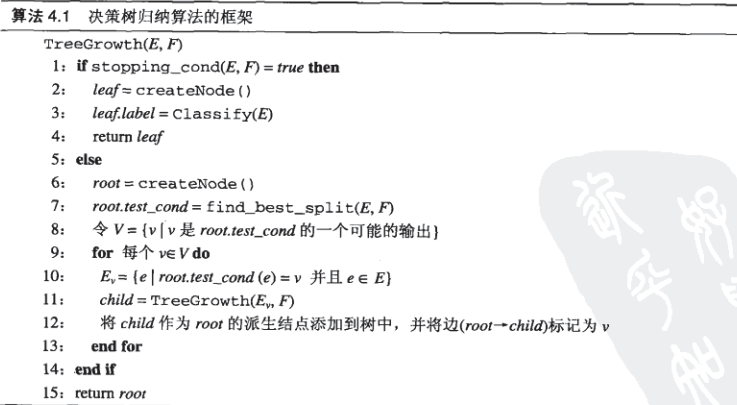2 程序设计

程序设计必须对算法的每个细节都要搞清楚，有时可能要实现一个健全完善的算法很困难，我们可以对算法进行简化，忽略复杂的情况，比如，在上面的构建决策树算法的步骤中，子树的划分可能有多个输出，连续属性和无序离散属性的划分的方法也有所不同，如果都要将这些考虑进去程序的设计难度会很大。作为初学者，可以对问题进行简化：

• 假设无序离散属性都只是二元属性，属性值用0或1表示
• 类别只有两类，用0或1表示
• 每个节点只有两个输出

在明确了细节之后，还需考虑另外一个问题：数据结构。在程序中用什么数据结构来描述所构建的“树”？这一步很关键，因为在对训练集之外的记录进行测试的时候要用到该数据结构。

由于自己实现决策树算法的目的只是加深对算法的理解，并不是实际开发，因此，只是将“树”的结构和参数打印出来。

function build_tree(x, y, L, level, parent_y, sig, p_value)
% 自编的用于构建决策树的简单程序，适用于属性为二元属性，二分类情况。（也可对程序进行修改以适用连续属性）。
% 输入：
% x：数值矩阵，样本属性记录（每一行为一个样本）
% y：数值向量，样本对应的labels
% 其它参数调用时可以忽略，在递归时起作用。
% 输出：打印决策树。
if nargin == 2
level = 0;
parent_y = -1;
L = 1:size(x, 2);
sig = -1;
p_value = [];
%        bin_f = zeros(size(x, 2), 1);
%        for k=1:size(x, 2)
%            if length(unique(x(:,k))) == 2
%               bin_f(k) = 1;
%            end
%        end
end
class = [0, 1];
[r, label] = is_leaf(x, y, parent_y); % 判断是否是叶子节点
if r
if sig ==-1
disp([repmat('     ', 1, level), 'leaf (', num2str(label), ')']);
elseif sig ==0
disp([repmat('     ', 1, level), '<', num2str(p_value),' leaf (', num2str(label), ')']);
else
disp([repmat('     ', 1, level), '>', num2str(p_value),' leaf (', num2str(label), ')']);
end
else
[ind, value, i_] = find_best_test(x, y, L); % 找出最佳的测试值
%
%         if ind ==1
%            keyboard;
%         end

[x1, y1, x2, y2] = split_(x, y, i_, value); % 实施划分
if sig ==-1
disp([repmat('     ', 1, level), 'node (', num2str(ind), ', ', num2str(value), ')']);
elseif sig ==0
disp([repmat('     ', 1, level), '<', num2str(p_value),' node (', num2str(ind), ', ', num2str(value), ')']);
else
disp([repmat('     ', 1, level), '>', num2str(p_value),' node (', num2str(ind), ', ', num2str(value), ')']);
end
%         if bin_f(i_) == 1
x1(:,i_) = [];
x2(:,i_) = [];
L(:,i_) = [];
%             bin_f(i_) = [];
%         end
build_tree(x1, y1, L, level+1, y, 0, value); % 地柜调用
build_tree(x2, y2, L, level+1, y, 1, value);
end

function [ind, value, i_] = find_best_test(xx, yy, LL) % 子函数：找出最佳测试值（可以对连续属性适用）
imp_min = inf;
i_ = 1;
ind = LL(i_);
for i=1:size(xx,2);
if length(unique(xx(:,i))) ==1
continue;
end
%            [xx_sorted, ii] = sortrows(xx, i);
%            yy_sorted = yy(ii, :);
vv = unique(xx(:,i));
imp_min_i = inf;
best_point = mean([vv(1), vv(2)]);
value = best_point;
for j = 1:length(vv)-1
point = mean([vv(j), vv(j+1)]);
[xx1, yy1, xx2, yy2] = split_(xx, yy, i, point);
imp = calc_imp(yy1, yy2);
if imp<imp_min_i
best_point = point;
imp_min_i = imp;
end
end
if imp_min_i < imp_min
value = best_point;
imp_min = imp_min_i;
i_ = i;
ind = LL(i_);
end
end
end

function imp = calc_imp(y1, y2) % 子函数：计算熵
p11 = sum(y1==class(1))/length(y1);
p12 = sum(y1==class(2))/length(y1);
p21 = sum(y2==class(1))/length(y2);
p22 = sum(y2==class(2))/length(y2);
if p11==0
t11 = 0;
else
t11 = p11*log2(p11);
end
if p12==0
t12 = 0;
else
t12 = p12*log2(p12);
end
if p21==0
t21 = 0;
else
t21 = p21*log2(p21);
end
if p22==0
t22 = 0;
else
t22 = p22*log2(p22);
end

imp = -t11-t12-t21-t22;
end

function [x1, y1, x2, y2] = split_(x, y, i, point) % 子函数：实施划分
index = (x(:,i)<point);
x1 = x(index,:);
y1 = y(index,:);
x2 = x(~index,:);
y2 = y(~index,:);
end

function [r, label] = is_leaf(xx, yy, parent_yy) % 子函数：判断是否是叶子节点
if isempty(xx)
r = true;
label = mode(parent_yy);
elseif length(unique(yy)) == 1
r = true;
label = unique(yy);
else
t = xx - repmat(xx(1,:),size(xx, 1), 1);
if all(all(t ==0))
r = true;
label = mode(yy);
else
r = false;
label = [];
end
end
end
end

利用MATLAB提供的数据集进行测试，并与 MATLAB 自身提供的决策树分类的函数进行对比。

clc
clear all
load ionosphere % contains X and Y variables
x = X(:,1:3);
ind = x(:,3)>0;
x(ind,3) = 1;
x(~ind,3) = 0;

y = zeros(size(Y));
y(ismember(Y, 'b')) = 1;

ctree = fitctree(x, y);
view(ctree,'mode','graph') % graphic description
% [label, score] = predict(ctree, X(5,:))

build_tree(x, y);

自编程序运行结果

含义说明：

node(属性序号， 划分点)

leaf(类别)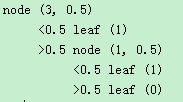MATLAB提供的函数的运行结果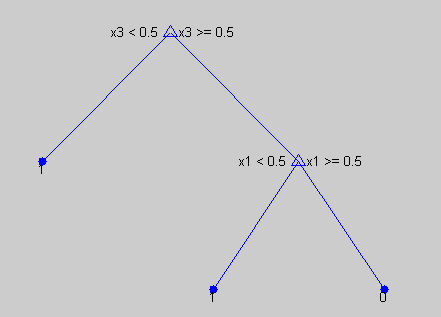结果与MATLAB中自己实现的函数运行结果相同。

3 MATLAB 中的调用

自己对算法的实现的目的主要还是用于加深对算法的理解，但是在实际应用时，还得借助成熟的机器学习工具包，比如MATLAB或Python提供的机器学习工具包。下面介绍一下MATLAB中决策树算法的相关函数的调用方法。

tree = fitctree(x,y)
tree = fitctree(x,y,Name,Value)

根据给定的记录的属性x，对应类别y，构造决策树（二叉树）。要求x为数值矩阵，y为数值向量或cell数组。name-value pair 为可选参数，用于指定算法的参数（划分准则，叶子节点最少记录值等）。x, y 每一行为一个样本。

返回tree为决策树的数据结构。

利用tree进行分类：

label = predict(tree, x)

4 Python 中的调用

scikit-learn 库提供了决策树分类和回归的方法.

训练

>>> from sklearn import tree
>>> X = [[0, 0], [1, 1]]
>>> Y = [0, 1]
>>> clf = tree.DecisionTreeClassifier()
>>> clf = clf.fit(X, Y)

分类

>>> clf.predict([[2., 2.]])
array()

DecisionTreeClassifier能够进行二元分类(标签为[- 1,1])和多类分类(标签为[0，…，K-1])。

展开全文healingwounds 2018-10-24 19:22:51
• 987KB weixin_38747946 2021-05-24 08:34:27
• weixin_35754962 2021-04-30 05:56:14
• 89KB weixin_38660813 2021-05-26 23:27:22
• kd树建立 MATLAB源代码 kdtree5星
6KB icream_lk 2012-05-05 13:31:15
• MATLAB机器学习系列-9：决策树和随机森林的原理及其例子代码实现 matlab 机器学习

kobeyu652453 2020-10-31 10:48:50
• 7KB weixin_38517728 2021-05-24 08:35:29
• 702KB weixin_38680492 2021-05-21 09:39:22
• 38KB weixin_38516658 2021-05-22 07:55:23
• 元胞自动机(CA)详解+Matlab代码实现 元胞自动机 matlab 数学建模 蒙特卡罗模拟

weixin_51545953 2021-11-25 12:56:07
• 20.92MB weixin_38596093 2021-05-21 17:55:12
• 3.23MB weixin_38728555 2021-05-21 10:55:41
• lishan132 2020-09-11 09:29:25
• 霍夫曼编译码的Matlab代码实现 二叉树 数据结构 算法

qq_40700822 2020-01-28 17:02:27
• MATLAB实现基本决策树(未剪枝) MATLAB 决策树 西瓜书

lyd18916269803 2019-09-29 09:51:24...

matlab树结构代码实现matlab 订阅